Problem

# A circular wire loop of radius a and resistance R initially has a magnetic flux through it...

A circular wire loop of radius a and resistance R initially has a magnetic flux through it due to an external magnetic field. The external field then decreases to zero. A current is induced in the loop while the external field is changing; however, this current does not stop at the instant that the external field stops changing. The reason is that the current itself generates a magnetic field, which gives rise to a flux through the loop. If the current changes, the flux through the loop changes as well, and an induced emf appears in the loop to oppose the change. (a) The magnetic field at the center of the loop of radius a produced by a current i in the loop is given by B = μ0i/2a. If we use the crude approximation that the field has this same value at all points within the loop, what is the flux of this field through the loop? (b) By using Faraday’s law, Eq. (29.3), and the relationship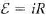, show that after the external field has stopped changing, the current in the loop obeys the differential equation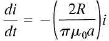(c) If the current has the value i0 at t = 0, the instant that the external field stops changing, solve the equation in part (b) to find i as a function of time for t > 0. (Hint: In Section 26.4 we encountered a similar differential equation, Eq. (26.15), for the quantity q. This equation for i may be solved in the same way.) (d) If the loop has radius a = 50 cm and resistance R = 0.10 Ω, how long after the external field stops changing will the current be equal to 0.010i0 (that is,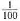of its initial value)? (e) In solving the examples in this chapter, we ignored the effects described in this problem. Explain why this is a good approximation.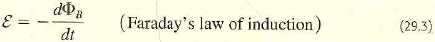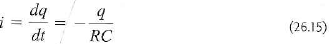#### Step-by-Step Solution

Request Professional Solution

#### Request solution!

We need 10 more requests to produce this problem solution. Share with your friends to get the problem solution faster!

0 /10 have requested this problem solution

Once 10 people have made a request, the problem solutions will be available in 1-2 days.
All students who have requested the problem solution will be notified once they are available.